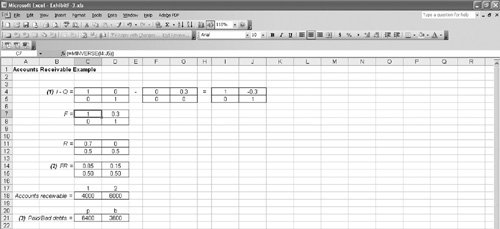# Excel Solution of the Debt Example[Page F-16 ( continued )]

All the matrix operations performed manually in the previous section for the debt example can be accomplished by using Excel. Exhibit F.3 shows an Excel spreadsheet with the matrix operations for our A-to-Z Office Supply Company accounts receivable example.

##### Exhibit F.3.[Page F-17]

In step 1 the fundamental matrix, F , is developed. First, the Q matrix is subtracted from the identity matrix, I. This can be done by inputting the numeric values from our example in the matrices set up in cells C4:D5 and F4:G5 , and subtracting the array in F4:G5 from the array in C4:D5 . This subtraction can be conveniently accomplished by covering cells I4:J5 with the cursor, embedding the formula =( C4:D5 ) ( F4:G5 ) in cell I4 ; then with the "Ctrl" and "Shift" keys pressed down, we press "Enter."

Next, we take the inverse of the matrix in cells I4:J5 . This is done by covering cells C7:D8 with the cursor and entering the formula shown on the formula bar at the top of the spreadsheet in cell C7. Then with the "Ctrl" and"Shift" keys pressed down, we press "Enter."

In step 2 the FR matrix is computed by entering the matrix operation formulas for multiplying two matrices in cells C14:D15 . For example, the formula = C7 * C11 + D7 * C12 is entered in cell C14, which results in the value 0.85 in cell C14.

Finally, in step 3 the matrix values indicating the amounts of paid and bad debts for our example are computed, using the formulas for multiplying two matrices. For example, the formula in cell C21 is = C14 * C18 + C15 * D18, resulting in the value 6,400.Introduction to Management Science (10th Edition)
ISBN: 0136064361
EAN: 2147483647
Year: 2006
Pages: 358

Similar book on Amazon

flylib.com © 2008-2017.
If you may any questions please contact us: flylib@qtcs.net